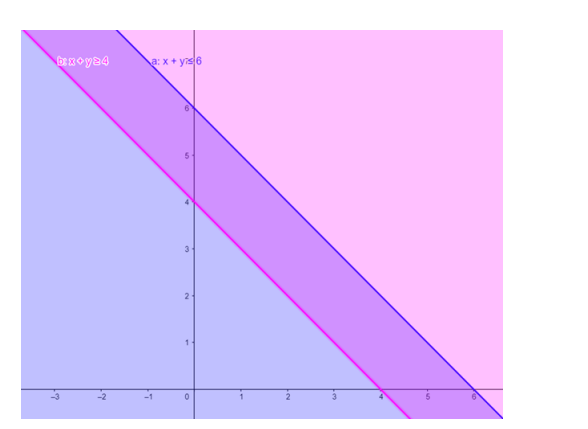# Solve the given inequalitiesQuestion:

Solve the given inequalities $x+y \leq 6, x+y \geq 4$ graphically in two-dimensional plane.

Solution:

The graphical representation of $x+y \leq 6, x+y \geq 4$ is given by common region in the figure below.

$x+y \leq 6 \ldots \ldots(1)$

$x+y \geq 4 \ldots \ldots(2)$

Inequality (1) represents the region below line $x+y=6$ (including the line $x+y=6$ ).

Inequality (2) represents the region above line $x+y=4$ (including the line $x+y=4$ ).

Therefore, every point in the common shaded region including the points on the respective lines represents the solution for the given inequalities.

This can be represented as follows,